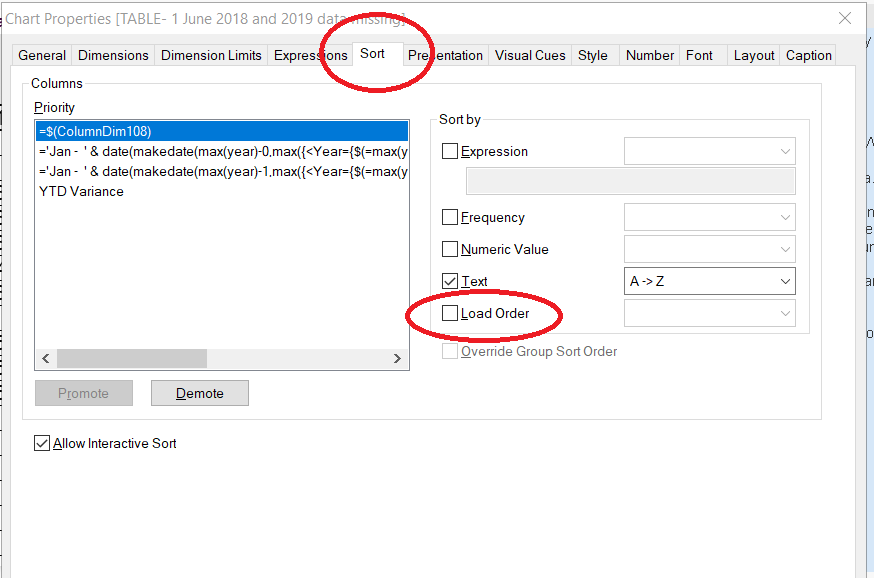# QlikView App Development

Discussion Board for collaboration related to QlikView App Development.

Announcements
Modernize Your QlikView Deployment webinar, Nov. 3rd. REGISTER
cancel
Showing results for
Did you mean:
HighlightedContributor III

## Sorting

Hi,

This is my Dimenssion
I want to sort my data with this dimenssion

=if(Aggr(sum(Gross_Sales),Year,Customer)<0,'Negative Sale',
if(Aggr(sum(Gross_Sales),Year,Customer)=0,'0 Sale',
if((Aggr(sum(Gross_Sales),Year,Customer)>0 and Aggr(sum(Gross_Sales),Year,Customer)<=500),'1-500',
if((Aggr(sum(Gross_Sales),Year,Customer)>500 and Aggr(sum(Gross_Sales),Year,Customer)<=1000),'501-1000',
if((Aggr(sum(Gross_Sales),Year,Customer)>1000 and Aggr(sum(Gross_Sales),Year,Customer)<=3000),'1001-3000',
if((Aggr(sum(Gross_Sales),Year,Customer)>3000 and Aggr(sum(Gross_Sales),Year,Customer)<=5000),'3001-5000',
if((Aggr(sum(Gross_Sales),Year,Customer)>5000 and Aggr(sum(Gross_Sales),Year,Customer)<=10000),'5001-10000',
if((Aggr(sum(Gross_Sales),Year,Customer)>10000 and Aggr(sum(Gross_Sales),Year,Customer)<=30000),'10001-30000',
if((Aggr(sum(Gross_Sales),Year,Customer)>30000 and Aggr(sum(Gross_Sales),Year,Customer)<=50000),'30001-50000',
if((Aggr(sum(Gross_Sales),Year,Customer)>50000 and Aggr(sum(Gross_Sales),Year,Customer)<=100000),'50001-100000',
if((Aggr(sum(Gross_Sales),Year,Customer)>100000 and Aggr(sum(Gross_Sales),Year,Customer)<=500000),'100001-500000',
if(Aggr(sum(Gross_Sales),Year,Customer)>500000,'500000+'

))))))))))))

19 Replies
HighlightedMaster II

HighlightedContributor III

Thanks but where is that option load order

please can you give me that screen shot

HighlightedContributor III

I found Load Order but for my window it is disable for me

what can i do

HighlightedMaster IIHighlightedMaster II

Do you have any option selected? If yes you can deselected and try

HighlightedMaster II

What kind of graph or table do you have selected?

HighlightedContributor III

I attached the image

HighlightedMaster II

Send me a picture of the sort tab

HighlightedContributor III

Here is the attached file of sort Tab